Smartick is a fun way to learn math!Mar19

# Do You Want to Learn the Properties of Powers?

In today’s post we’re going to talk about some of the properties found in powers.

But first it’ll be useful to review the concept of a power, its elements, and how to use it. You can find all this in another Smartick blog post.

Once you’ve finished recapping, we can carry on.

### Powers with an exponent of 0

These powers are those that have an integer as a base and a zero or neutral element as an exponent. They are always equal to one.Take a look at this second example: 2 to the power of 0 equals 1.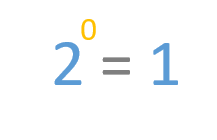### Powers with an exponent of 1

These powers have an integer as a base and 1 as their exponent. They are always equal to the base without an exponent. It seems obvious once you know it, but it’s an interesting peculiarity involving the powers.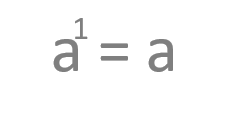Let’s have a look at an example involving a number: 2 to the power of 1 equals 2.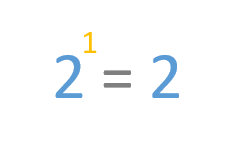### Products of powers with the same base

In this case, we’re going to look at what happens when we multiply two powers that have the same base (a) but different exponents (n and m). This expression can be simplified into a power with the same base and a new exponent, which is the result of adding the existing exponents (n + m).Let’s look at an example. If we multiply 2 to the power of 2 by 2 to the power of 3 we can see it’s the same as 2 to the power of 5.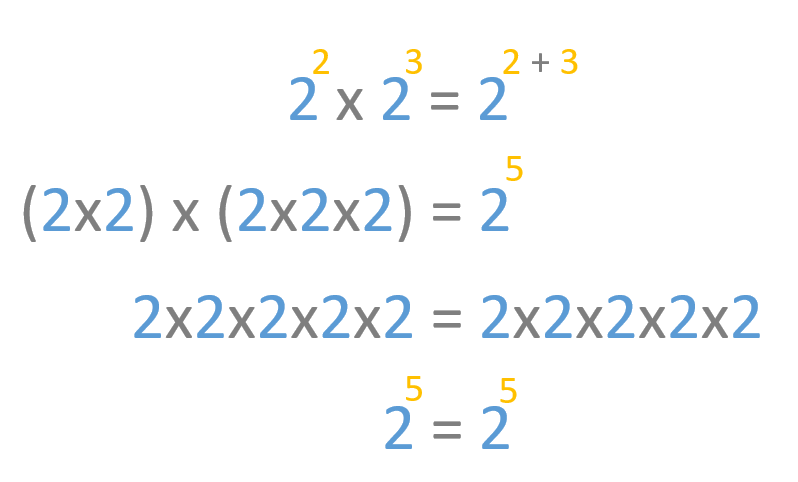### Products of division with the same base

Here we’re going to see what happens when we divide two powers that have the same base (a) but different exponents (n and m). This expression can be simplified by subtracting the exponent of the dividend from the exponent of the divisor (n – m), with the result giving us the exponent of the result with the same base.Here’s an example. We divide 2 to the power of 4 by 2 to the power of 2 and we can see the result is the same as 2 to the power of 2.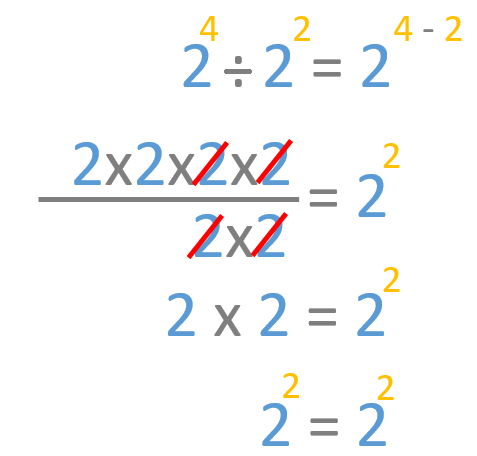I hope this post has helped you to better understand the properties and peculiarities of powers. Keep checking back for the next post on the rest of their properties!

Remember, if you want to practice with more primary math exercises, you’ll find them in our daily sessions. Log in to Smartick and try our online math learning method for free.

Fun is our brain’s favorite way of learning
Diane Ackerman
Smartick is a fun way to learn math
• 15 fun minutes a day